MathScore EduFighter is one of the best math games on the Internet today. You can start playing for free!

## Triangle Angles 2 - Sample Math Practice Problems

The math problems below can be generated by MathScore.com, a math practice program for schools and individual families. References to complexity and mode refer to the overall difficulty of the problems as they appear in the main program. In the main program, all problems are automatically graded and the difficulty adapts dynamically based on performance. Answers to these sample questions appear at the bottom of the page. This page does not grade your responses.

See some of our other supported math practice problems.

### Complexity=5, Mode=numSupp

Find the value of 'x'. Diagrams are not always to scale.

 1.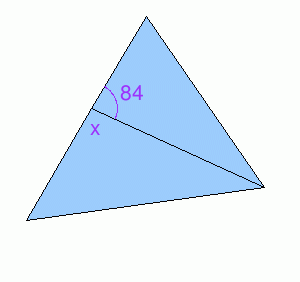x = 2.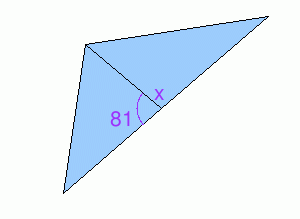x =

### Complexity=8, Mode=varSupp

Find the value of 'x'. Diagrams are not always to scale.

 1.x = 2.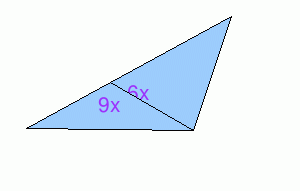x =

### Complexity=10, Mode=distAng

Find the value of 'x'. Diagrams are not always to scale.

 1.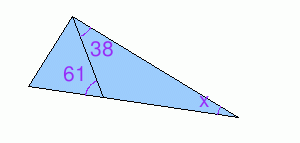x = 2.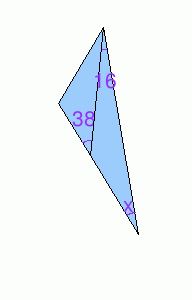x =

### Complexity=20

Find the value of 'x'. Diagrams are not always to scale.

 1.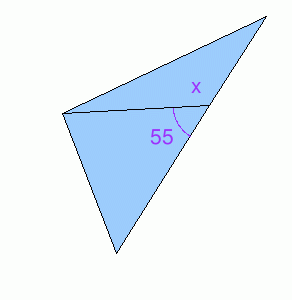x = 2.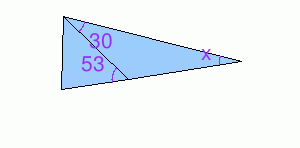x =

### Complexity=5, Mode=numSupp

Find the value of 'x'. Diagrams are not always to scale.

1x =
Solution
The two angles form a line so they are supplementary angles.
The sum of supplementary angles is 180 degrees.
84 + x = 180
x = 180 - 84
x = 96
2x =
Solution
The two angles form a line so they are supplementary angles.
The sum of supplementary angles is 180 degrees.
81 + x = 180
x = 180 - 81
x = 99

### Complexity=8, Mode=varSupp

Find the value of 'x'. Diagrams are not always to scale.

1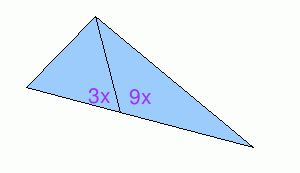x =
Solution
The two angles form a line so they are supplementary angles.
The sum of supplementary angles is 180 degrees.
3x + 9x = 180
12x = 180
12x ÷ 12 = 180 ÷ 12
x = 15
2x =
Solution
The two angles form a line so they are supplementary angles.
The sum of supplementary angles is 180 degrees.
6x + 9x = 180
15x = 180
15x ÷ 15 = 180 ÷ 15
x = 12

### Complexity=10, Mode=distAng

Find the value of 'x'. Diagrams are not always to scale.

1x =
Solution
The angle marked as 61 degrees forms a line with the angle next to it. They are supplementary angles.
Let y be this supplementary angle.
61 + y = 180
y = 180 - 61 = 119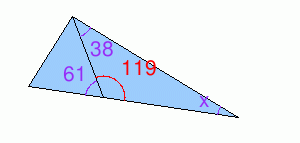The angles of a triangle add up to 180 degrees..
x + 119 + 38 = 180
x + 157 = 180
x = 23
2x =
Solution
The angle marked as 38 degrees forms a line with the angle next to it. They are supplementary angles.
Let y be this supplementary angle.
38 + y = 180
y = 180 - 38 = 142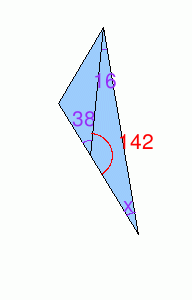The angles of a triangle add up to 180 degrees..
x + 142 + 16 = 180
x + 158 = 180
x = 22

### Complexity=20

Find the value of 'x'. Diagrams are not always to scale.

1x =
Solution
The two angles form a line so they are supplementary angles.
The sum of supplementary angles is 180 degrees.
55 + x = 180
x = 180 - 55
x = 125
2x =
Solution
The angle marked as 53 degrees forms a line with the angle next to it. They are supplementary angles.
Let y be this supplementary angle.
53 + y = 180
y = 180 - 53 = 127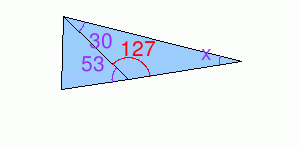The angles of a triangle add up to 180 degrees..
x + 127 + 30 = 180
x + 157 = 180
x = 23